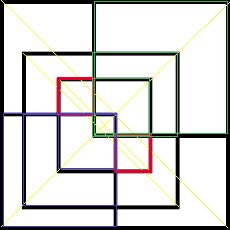# How Many Squares?

How many squares are in this image?

But here’s the twist: you have to present your logic behind the answer.

There are a total of 12 squares.
How?
Actually finding number of squares in this picture is a little tricky, I would recommend you to take a print out or open the image in Microsoft paint.
Basically idea is to draw diagonals rectangles, now as sides of the squares are parallel (either horizontal or vertical) so diagonals should also be parallel, as the outer most rectangle is nothing but square we should take this as a reference.
I have drawn few yellow lines as diagonals for reference.Number of Squares in above image (marked with red): 4 (we will consider other rectangles in next images).Number of Squares in above image: 3(in blue) + 3(in green) + 2(in red) (other 4 rectangles are not squares as their diagonal is not parallel to the yellow lines.)
Thus, total number of squares: 4+3+3+2= 12